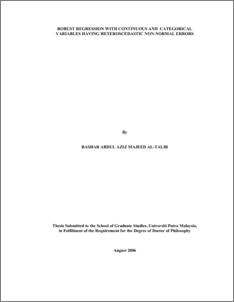# Robust Regression with Continuous and Categorical Variables Having Heteroscedastic Non-Normal Errors

## Citation

Majeed Al-Talib, Bashar Abdul Aziz (2006) Robust Regression with Continuous and Categorical Variables Having Heteroscedastic Non-Normal Errors. PhD thesis, Universiti Putra Malaysia.

## Abstract

The performance of the classical Ordinary Least Squares (OLS) method can be very poor when the data set for which one often makes a normal assumption, has a heavy- tailed distribution which may arise as a result of outliers. The problem is further complicated when the variances of the error terms are not constant. In this thesis, a Reweighted Least Squares based on Robust Distance and a combination between the S and M-estimates (called S/M Estimates). The resulted estimates will be called (RLSRDSM) estimates, which proposed to overcome the problem of outlier. The RLSRDSM estimates are proposed to estimates the parameters of a regression model with both continuous and categorical variables. The Robust Distance S/M Estimates are computed in three stages where on the first stage the Minimum Volume Ellipsoid (MVE) estimator is computed to identify leverage points, then a weighted S/M weights and scale is calculated in the second and third steps respectively. In many applications, one may encounter errors which are heteroscedastic and not normally distributed. Therefore, in this thesis, a weighted RLSRDSM (WRLSRDSM) is proposed to remedy these two problems simultaneously. This method first computes the residuals scale estimates for each level of the categorical variables based on RDSM residuals. A weighted scheme is then developed and incorporates in the model. In addition to RLSRDSM and WRLSRDSM, another estimator which is referred as 2D-RDLS procedure that use two-dimensional weighting scheme is also proposed. However, the performance of the 2D-RDLS estimates is not as good as the RLSRDSM and therefore seldom referred in the discussion. A number of numerical examples and simulation studies have been performed to compare the robustness of the RLSRDSM, and WRLSRDSM with some existing methods in the regression model with both continuous and categorical regressors. Data with various outlier contamination were simulated and analyzed. Design parameters were varied, include sample size (n=20, 50, 100, 300, and 500), number of continuous regressors (p=1,3, and 5) and categorical data (q=1,4), outliers density (0%, 5%, 10%, 20%, 30%, 40%, and 50%), and different error distribution scenarios (N(0,0.25), N(0,0.5), N(0,1), N(0,2), N(0,3), N(0,4), t(3), and EXP(1)). Criteria used to measure the performance of the regression methods are p-values, residual scale, %, and %for the real data analysis and The Root Mean Square Error (RMSE) of the overall simulation replications which summarized the variance and bias for the simulated data. The results in this thesis indicate that the Ordinary Least Squares (OLS) estimators are very sensitive to the presence of outliers and heteroscedastic errors. In the presence of outliers, the RLSRDSM and RLSRDL1 are better than OLS, by producing robust estimates to such kind of data points. The RLSRDSM is slightly better than RLSRDL1 and sometimes their performances are indistinguishable in the presence of outliers. Nonetheless, the RLSRDL1 posed certain computational problems such as producing degenerate solution or singular matrices. The advantage of RLSRDSM is that it has no computational problems. The performance of WRLSRDSM is better than the WLSRDL1 when both outliers and heteroscedastics occurs together. In order to support the numerical findings, Bootstrap simulation procedures and visual analysis are also been carried out to justify that the RLSRDSM is the most robust estimator compared to the OLS and RLSRDL1, on a ground that this estimator result with robust and stable in the presence of outliers, combined models with continuous and categorical variables, and even heteroscedasticity problem. The results indeed show that they are in close agreement with the earlier conclusion.Preview
PDF
600391_fs_2006_52_abstrak_je__dh_pdf_.pdfView Item